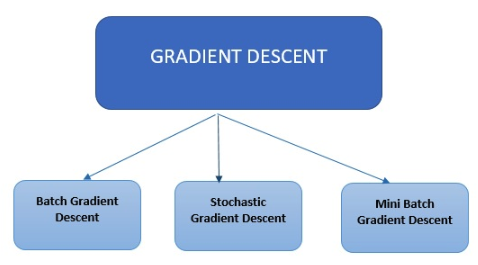Gradient descent is the most commonly used optimization method deployed in machine learning and deep learning algorithms. It’s used to train a machine learning model.There are three primary types of gradient descent used in modern machine learning and deep learning algorithms.

Batch Gradient Descent is the most straightforward type. It calculates the error for each example in the training dataset, however, it only updates the model after all training examples have been evaluated.

Stochastic Gradient Descent calculates the error and updates the model for each example in the training dataset.